Courses

# Test: Degree Of Dissociation And Vapour Density(Chemical Equilibrium)

## 15 Questions MCQ Test Chemistry for JEE | Test: Degree Of Dissociation And Vapour Density(Chemical Equilibrium)

Description
This mock test of Test: Degree Of Dissociation And Vapour Density(Chemical Equilibrium) for JEE helps you for every JEE entrance exam. This contains 15 Multiple Choice Questions for JEE Test: Degree Of Dissociation And Vapour Density(Chemical Equilibrium) (mcq) to study with solutions a complete question bank. The solved questions answers in this Test: Degree Of Dissociation And Vapour Density(Chemical Equilibrium) quiz give you a good mix of easy questions and tough questions. JEE students definitely take this Test: Degree Of Dissociation And Vapour Density(Chemical Equilibrium) exercise for a better result in the exam. You can find other Test: Degree Of Dissociation And Vapour Density(Chemical Equilibrium) extra questions, long questions & short questions for JEE on EduRev as well by searching above.
QUESTION: 1

### Direction (Q. Nos. 1-8) This section contains 8 multiple choice questions. Each question has four choices (a), (b), (c) and (d), out of which ONLY ONE option is correct. Q. For the following equilibrium, PCl5(g)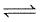PCl3(g) + Cl2 (g) Vapour density is found to be 100 when 1 mole of PCI5 is taken in 10 dm3 flask at 300 K. Thus, equilibrium pressure is

Solution:

Given, vapour pressure = 100
So, molecular weight = 200
Degree of dissociation;α = 1/(n-1)[Mtheo-Mobs/Mobs]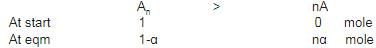α   =   1/(2-1)×[208.5-200/208.5]
α  = 0.0425
PCl5(s)    ⇌    PCl3(g) + Cl2(g)
At start      1                 -           -     -
At eqm.  (1-0.0428)   (0.0428)  (0.0428)
Total moles at eqm. = 1.0428
Total pressure at equilibrium; pV = nRT
p = 1.0428×0.0821×300/10
p  = 2.5676 atm

QUESTION: 2

Solution:
QUESTION: 3

### In the following equilibrium at 400 K in 1 dm3 flask, COCl2 (g)CO(g) + Cl2 (g), ratio of theoretical and experimental vapour density is 1.4. Thus, equilibrium constant Kc is

Solution:
QUESTION: 4

A(g) is 100% converted into B according to the reaction,
A(g)3b(g)
Value of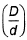at this point is

Solution:

A(g)↔3B(g)
t  = 0               1             0
t1 = t1                      −x          +3x
at eq=teq                 1−x         3x
Given that x=0.90
Total moles at equilibrium= 1−x+3x = 1+2x
= 1+2(0.90) = 2.8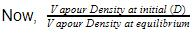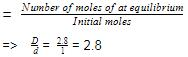QUESTION: 5

In the dissociation of PCI5(g)into PCI3(g)and Cl2(g), values of(1 + x)have been plotted versus D/d. Thus, correct graph is

Solution:
QUESTION: 6

CCI4(g) dissociates 50% into gaseous free radicals at 1000 K and at equilibrium experimental vapour density is found to be 38.5. Thus, CCI4(g) dissociates under the given conditions to

Solution:
QUESTION: 7

0.578 g N2O4(g) dissociates into 1dm3 flask at 308 K to give a pressure of 24.12 kPa.
N2O4 (g)2NO2(g)
Thus, degree of dissociation of N2O4 is

Solution:
QUESTION: 8

SO3(g)is partially dissociated into SO2(g)and O2(g) at 900K and 1 atm as: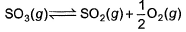The density of the equilibrium mixture was found to be 0.866 gdm-3. Thus, degree of dissociation of SO3 (g) is

Solution:
QUESTION: 9

Direction (Q. Nos. 9-12) This section contains  a paragraph, wach describing theory, experiments, data etc. three Questions related to paragraph have been given.Each question have only one correct answer among the four given options (a),(b),(c),(d)

Passage I

Vapour density of the equilibrium mixture of NO2 and N2O4 is found to be 40 for the gaseous phase equilibrium at 400 K in 1 dm3 flask.
N2O4 (g)2NO2(g)

Q. Equilibrium Constant Kc is

Solution:
QUESTION: 10

Passage I

Vapour density of the equilibrium mixture of NO2 and N2O4 is found to be 40 for the gaseous phase equilibrium at 400 K in 1 dm3 flask.

N2O4 (g)2NO2(g)

Q. Percentage of N02 in the mixture by volume is

Solution:
QUESTION: 11

Passage II

COCI2 (phosgene) gas dissociates according to

COCl2 (g)CO(g) + Cl2 (g)

At 101.325 kPa and at 724 K density of the gas mixture is 1.162 g dm-3.

Q. Observed molar mass of the gaseous mixture is

Solution:
QUESTION: 12

Passage II

COCI2 (phosgene) gas dissociates according to
COCl2 (g)CO(g) + Cl2 (g)

At 101.325 kPa and at 724 K density of the gas mixture is 1.162 g dm-3.

Q. Equilibrium constant Kp is

Solution: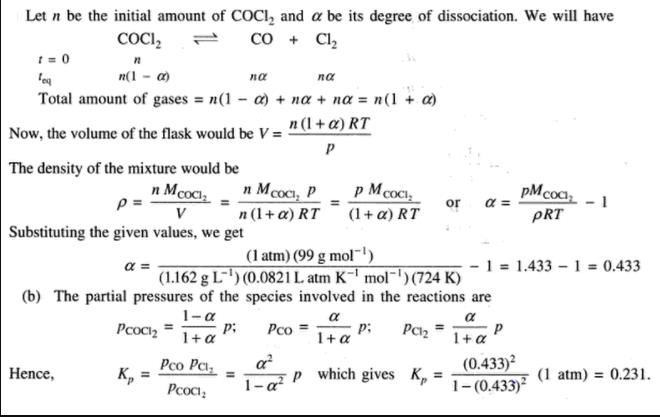*Answer can only contain numeric values
QUESTION: 13

Direction (Q. Nos. 21 and 22) This section contains 2 questions. when worked out will result in an integer from 0 to 9 (both inclusive)

Q. In the following hypothetical equilibrium,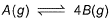Values of D/d (along y-axis) have been plotted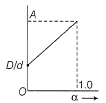versus a (along x-axis.)
α = degree of dissociation
d = experimental vapour density
D = theoretical vapour density

Q. What is the value of D/d at the point A corresponding to α = 1 ?

Solution:
QUESTION: 14

Direction (Q, Nos. 14 - 15) These questions are subjective in nature, need to be solved completely on notebook.

NO2 is 40.0% of the total volume at 300 K in the following equilibrium,

N2O4 (g)2NO2(g)

Calculate the ratio of vapour density in the absence and presence of dissociation.

Solution:
QUESTION: 15

PCI5(g) dissociates according to the reaction at 523 K.

PCl5(g)PCl3(g) + Cl2 (g)          KP = 1.78 atm
Compute the density of the equilibrium mixture in g dm-3 at 1 atm pressure.

Solution:

Correct answer is Option A.

PCl5   ⇌    PCl3   +    Cl2
Po(1-α)           Poα               Poα

​1.78     =     Poα2 /(1-α)
Po(1-α) =     1
Mavg     =    208.5 / (1+α)
Dmix       =    [1×208.5/ (1+α)] / [0.0821×523]
=    2.71 g/l
=    2.71 g/dm3
Hence, the density of the equilibrium mixture is 2.7gdm-3